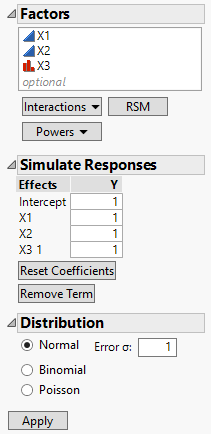Publication date: 03/23/2021

## Simulate Responses

When you click Make Table to create your design table, the Simulate Responses option does the following for each response:

It adds random response values to the response column in your design table.

It adds a new a column containing a simulation model formula to the design table. The formula and values are based on a main effects model.

A Model window opens where you can add and remove effects to define a model, specify parameter values, and select a response distribution for simulation. When you click Apply in the Model window, each column containing a simulation model formula is updated.

#### Control Window

Figure 7.21 shows the Model window for a design with two continuous factors (X1 and X2) and one two-level categorical factor (X3).

Figure 7.21 Simulate Responses Control WindowThe window has three outlines:

Factors

Simulate Responses

Distribution

The initial Simulate Responses outline shows terms for a main effects model with values of 1 for all coefficients. The Distribution outline shows a Normal distribution with error standard deviation equal to 1. If you have set Anticipated Coefficients as part of Power Analysis under Design Evaluation in the DOE window, then the initial values in the Simulate Responses outline are the values that you specified as Anticipated Coefficients and Anticipated RMSE (Error Std) in the Power Analysis outline.

#### Factors

Add terms to the simulation model using the Factors outline.

Interactions

Select factors in the list. Then select the interaction order from the Interactions menu. Those interactions are added to the list of Effects in the Simulate Responses outline.

RSM

Adds all possible response surface terms to the list of Effects in the Simulate Responses outline.

Powers

Select factors in the list. Then select the order from the Powers menu. Those powers are added to the list of Effects in the Simulate Responses outline.

#### Simulate Responses

To specify a model for simulated values, do the following:

1. For each term in the list of Effects, enter coefficients for the linear model used to simulate the response values. These define a linear function, L(x, β) = xβ. See the Simulate Responses outline in Figure 7.21:

The vector x consists of the terms that define the effects listed under Effects.

The vector β is the vector of model coefficients that you specify under Y.

2. Under Distribution, select a response distribution.

3. Click Apply. A <Y> Simulated column containing simulated values and their formula is added to the design table, where Y is the name of the response column.

Reset coefficients

Sets all coefficients to 0.

Remove Term

Remove terms from the list of Effects. Select the effects to remove and click Remove Term. Note that you cannot remove main effects.

#### Distribution

Choose from one of the available distributions in the Simulate Responses window:

Normal

Simulates values from a normal distribution. Enter a value for Error σ, the standard deviation of the normal error distribution. If you have designated factors to have Changes of Hard in the Factors outline, you can enter a value for Whole Plots σ, the whole plot error. If you have designated factors to have Changes of Hard and Very Hard, you can enter values for both the subplot and whole plot errors. When you click Apply, random values and a formula containing a random response vector based on the model are entered in the column <Y> Simulated.

Binomial

Simulates values from a binomial distribution. Enter a value for N, the number of trials. Random integer values are generated according to a binomial distribution based on N trials with probability of success 1/(1 + exp(-L(x, β))). When you click Apply, random values and their formula are entered in the column <Y> Simulated. A column called N Trials that contains the value N is also added to the data table.

Poisson

Simulates random integer values according to a Poisson distribution with parameter exp(L(x, β)). When you click Apply, random values and their formula are entered in the column <Y> Simulated.

Note: You can set a preference to simulate responses every time you click Make Table. To do so, select File > Preferences > Platforms > DOE. Select Simulate Responses.

Want more information? Have questions? Get answers in the JMP User Community (community.jmp.com).